## LSTM预测比特币价格## 入门概念

### 什么是 LSTM？

**长期记忆（LSTM）**是一种 RNN，它使我们不仅可以处理单个数据点（例如图像），还可以处理整个数据序列（例如语音或视频）。它们是时间序列预测的理想选择，它们是我们今天将要使用的架构类型。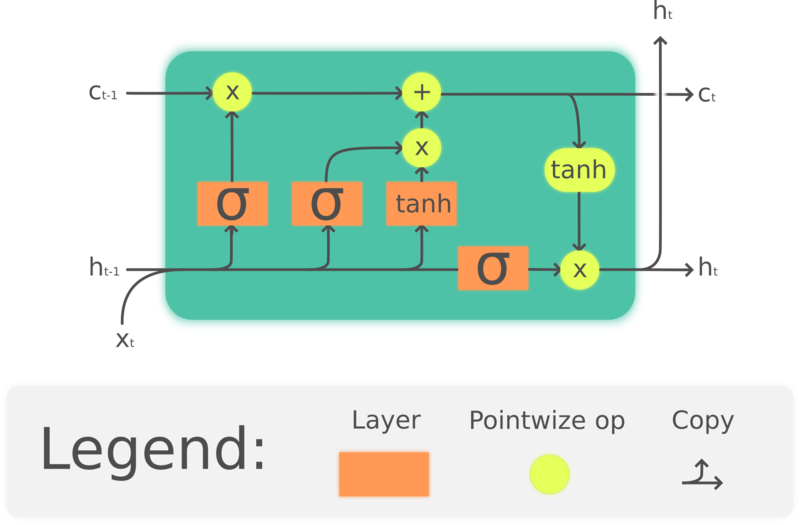LSTM 电池

## 数据探索

``````data = pd.read_csv("data/bitcoin.csv")
data = data.sort_values('Date')
``````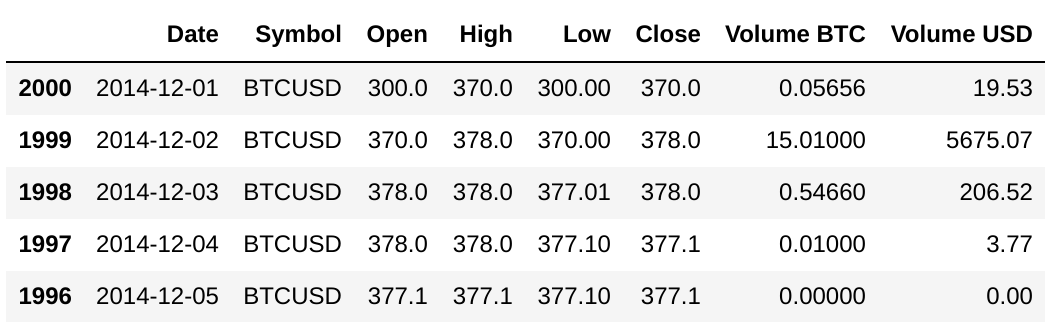`head()` 功能已经为我们提供了有关数据集的列以及这些信息的外观的一些有价值的信息。出于我们的目的，我们对该 `Close` 列感兴趣，该列包含该特定日期当天结束时的比特币价格。让我们看看是否可以使用我们的数据集构建一个图表，向我们显示比特币随时间的价格。

``````price = data[['Close']]

plt.figure(figsize = (15,9))
plt.plot(price)
plt.xticks(range(0, data.shape,50), data['Date'].loc[::50],rotation=45)
plt.title("Bitcoin Price",fontsize=18, fontweight='bold')
plt.xlabel('Date',fontsize=18)
plt.ylabel('Close Price (USD)',fontsize=18)
plt.show()
``````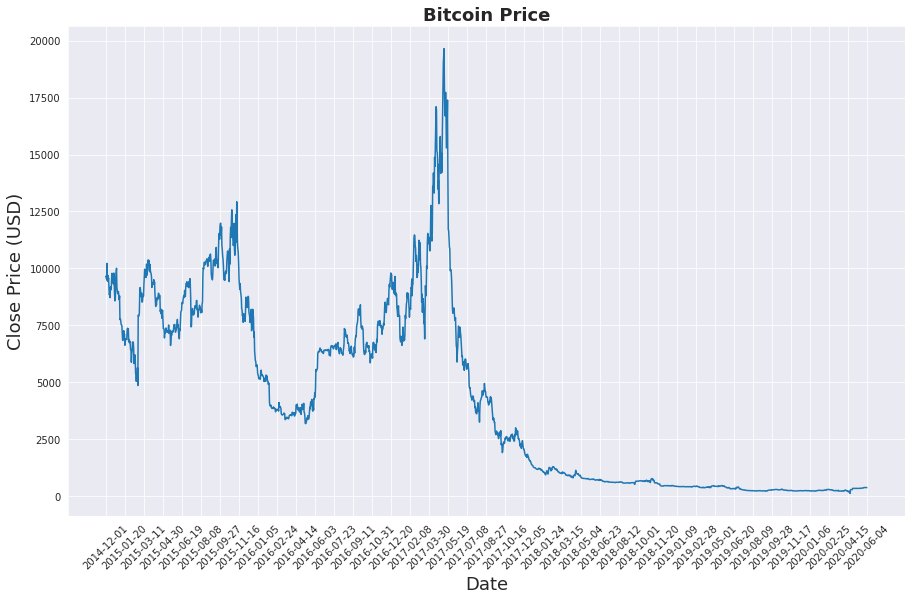2014 年 12 月至 2020 年 6 月的比特币价格

``````price.info()
<class 'pandas.core.frame.DataFrame'>
Int64Index: 2001 entries, 2000 to 0
Data columns (total 1 columns):
#   Column  Non-Null Count  Dtype
---  ------  --------------  -----
0   Close   2001 non-null   float64
dtypes: float64(1)
memory usage: 31.3 KB
``````

## 资料准备

### 正常化

``````from sklearn.preprocessing import MinMaxScaler
min_max_scaler = MinMaxScaler()

norm_data = min_max_scaler.fit_transform(price.values)
``````

``````Real: [370.], Normalized: [0.01280082]
Real: [426.1], Normalized: [0.01567332]
Real: [8259.99], Normalized: [0.41679416]
``````

### 数据分割

``````def univariate_data(dataset, start_index, end_index, history_size, target_size):
data = []
labels = []

start_index = start_index + history_size
if end_index is None:
end_index = len(dataset) - target_size

for i in range(start_index, end_index):
indices = range(i-history_size, i)
# Reshape data from (history_size,) to (history_size, 1)
data.append(np.reshape(dataset[indices], (history_size, 1)))
labels.append(dataset[i+target_size])
return np.array(data), np.array(labels)
``````

``````past_history = 5
future_target = 0

TRAIN_SPLIT = int(len(norm_data) * 0.8)

x_train, y_train = univariate_data(norm_data,
0,
TRAIN_SPLIT,
past_history,
future_target)

x_test, y_test = univariate_data(norm_data,
TRAIN_SPLIT,
None,
past_history,
future_target)
``````

## 建立模型

``````from keras.models import Sequential
from keras.layers import Dense, LSTM, LeakyReLU, Dropout

num_units = 64
learning_rate = 0.0001
activation_function = 'sigmoid'
loss_function = 'mse'
batch_size = 5
num_epochs = 50

# Initialize the RNN
model = Sequential()
model.add(LSTM(units = num_units, activation=activation_function, input_shape=(None, 1)))

# Compiling the RNN
``````

``````Model: "sequential_13"
_______________________________________________________________
Layer (type)                 Output Shape              Param #
===============================================================
lstm_6 (LSTM)                (None, 64)                16896
_______________________________________________________________
leaky_re_lu_4 (LeakyReLU)    (None, 64)                0
_______________________________________________________________
dropout_4 (Dropout)          (None, 64)                0
_______________________________________________________________
dense_6 (Dense)              (None, 1)                 65
===============================================================
Total params: 16,961
Trainable params: 16,961
Non-trainable params: 0
_______________________________________________________________
``````

## 训练模型

``````# Using the training set to train the model
history = model.fit(
x_train,
y_train,
validation_split=0.1,
batch_size=batch_size,
epochs=num_epochs,
shuffle=False
)
``````

``````loss = history.history['loss']
val_loss = history.history['val_loss']

epochs = range(len(loss))

plt.figure()

plt.plot(epochs, loss, 'b', label='Training loss')
plt.plot(epochs, val_loss, 'r', label='Validation loss')
plt.title("Training and Validation Loss")
plt.legend()

plt.show()
``````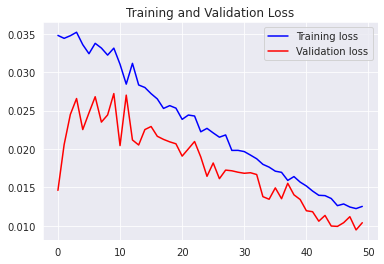## 预测

``````original = pd.DataFrame(min_max_scaler.inverse_transform(y_test))
predictions = pd.DataFrame(min_max_scaler.inverse_transform(model.predict(x_test)))

ax = sns.lineplot(x=original.index, y=original, label="Test Data", color='royalblue')
ax = sns.lineplot(x=predictions.index, y=predictions, label="Prediction", color='tomato')
ax.set_title('Bitcoin price', size = 14, fontweight='bold')
ax.set_xlabel("Days", size = 14)
ax.set_ylabel("Cost (USD)", size = 14)
ax.set_xticklabels('', size=10)
``````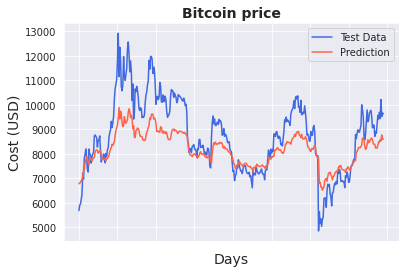## 结论

RNN 和 LSTM 是伟大的架构，我们可以用来分析和预测时间序列信息。在本文中，我们将重点更多地放在故事上，而不是实现的技术细节上，但是，如果您对该主题感兴趣，请进行研究，检查代码，使用它，更改层，超参数，尝试不同的事物，使用不同的专栏或规范化方法，阅读更详细的文章以及有关该主题的论文。